# Running Operations for Vectors

Calculates running functions with varying window size and missing handling options. Package brings also running streak and running which, what extends beyond range of functions already implemented in R packages. Calculating on windows depending on date also available.

# sportPackage contains standard running functions (aka. windowed, rolling, cumulative) with additional options. `runner` provides extended functionality like date windows, handling missings and varying window size. `runner` brings also rolling streak and rollin which, what extends beyond range of functions already implemented in R packages.

## Installation

Install package from from github or from CRAN.

## Examples

The main idea of the package is to provide running operations on R vectors. Running functions are these which are applied to all elements up to actual one. For example implemented already in `base` `cumsum`, `cummin` etc. Functions provided in this package works similar but with extended functionality such as handling `NA` and custom window size. The most functions provided in package are based on the same logic:

• window size `k` denotes number of elements from i-th backwards, where functions are calculated. `k` can be also specified by vector of the same length as x as illustrated in second example.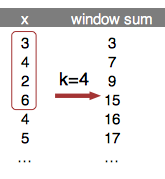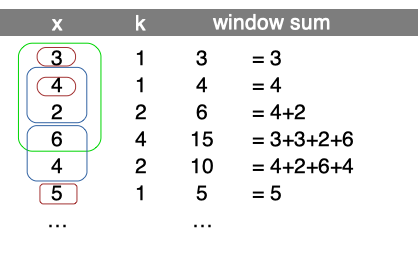• argument `na_rm=T` handling missing and is equivalent to `na.rm`.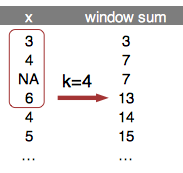• `na_pad=T` if window size exceeds number of available elements, than first `k-1` elements are filled with `NA`.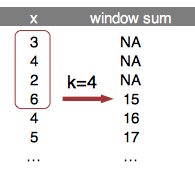• `which` used with running index, which value ('first' or 'last')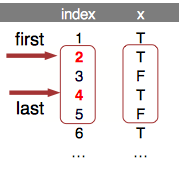• `idx` to calculate within time window. `idx` is a date (or numeric) vector of the same length as x. `k` then should to be an integer specyfying time window span. In the example k=5 window sum.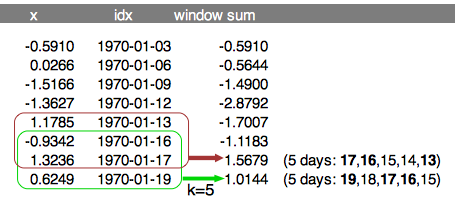### Creating windows

Function creates list of windows. Because `runner` provide limited functionality, one can create running-window-list which can be further processed by user to obtain desired statistic (eg. window sum). `x` is a vector to be 'run on' and `k` is a length of window. In this example window length is varying as specified by `k`. Provide one value to obtain constant window size.

Such windows can be used in further calculations, with any R function. Example below shows how to obtain running `sum` in specified, varying window length (specified by `k`).

One can also specify window based on a date of other numeric index. To do this date should be passed via `idx` argument and k should be integer denoting date-window span.

### Unique elements in window

User can use `unique_run` create list of unique elements within specified window size.

### Running aggregations `(mean|sum|min|max)_run`

Runner provides basic aggregation methods calculated within running windows. Below example showing some functions behavior for different arguments setup. Let's take a look at 8th element of a vector on which `min_run` is calculated. First setup uses default values, so algorithm is looking for minimum value in all elements before actual (i=8). By default missing values are removed before calculations by argument `na_rm=TRUE`, and also window is not specified. The default is equivalent of `base::cummin` with additional option to ignore `NA` values. In second example within window k=5, the lowest value is -3. In the last example minimum is not available due to existence of `NA`. Graphical example is reproduced below in the code.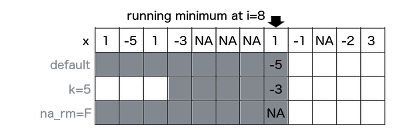### fill_run

Function used to replace `NA` with previous non-NA element. To understand how `fill_run` works, take a look on illustration. Row 'x' represents, and another rows represent replaced `NA` by fill_run with different options setup (run_for_first=TRUE and only_within=TRUE respectively). By default, `fill_run` replaces all `NA` if they were preceded by any value. If `NA` appeared in the beginning of the vector then it would not be replaced. But if user specify `run_for_first=TRUE` initial empty values values will be replaced by next non-empty value. Option `only_within=TRUE` means that `NA` values would be replaced if they were surrounded by pair of identical values. No windows provided in this functionality.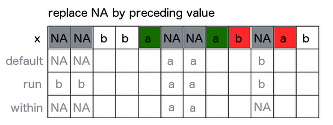### Running streak

To count consecutive elements in specified window one can use `streak_run`. Following figure illustrates how streak is calculated with three different options setup for 9th element of the input vector `x`. First shows default configuration, with full window and `na_rm=T`. Second example count within k=4 window with count reset on `NA`. Last example counting streak with continuation after `NA`. Visualization also supported with corresponding R code.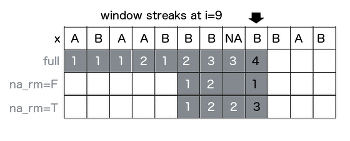### Running which

To obtain index number of element satisfying some condition in window, one can use running which. Functions from this group returns index while condition is `TRUE` appeared before n-th element of a vector. In below example `whicht_run` function returns index of element containing `TRUE` value. If `na_rm=T` is specified, missing is treated as `FALSE`, and is ignored while searching for `TRUE`. While user set `na_rm=F` like in second example, function returns `NA`, because in following window `TRUE` appears after missing and it's impossible to be certain which is first (missing is an element of unknown value - could be TRUE or FALSE).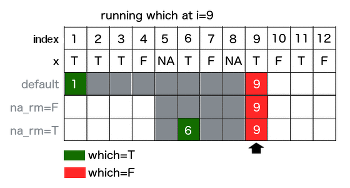# runner 0.2.0

## Improvements

• all functions have additional `idx` argument which allows to compute running windows within specified date/time/indexes range.
• added lag_run function - lagged value able to be computed on date/time lag.
• added length_run function to compute number of elements within specified date/time/indexes range.

# Reference manual

install.packages("runner")

0.3.2 by Dawid Kałędkowski, 20 days ago

Report a bug at https://github.com/gogonzo/runner/issues

Browse source code at https://github.com/cran/runner

Authors: Dawid Kałędkowski [aut, cre]

Documentation:   PDF Manual